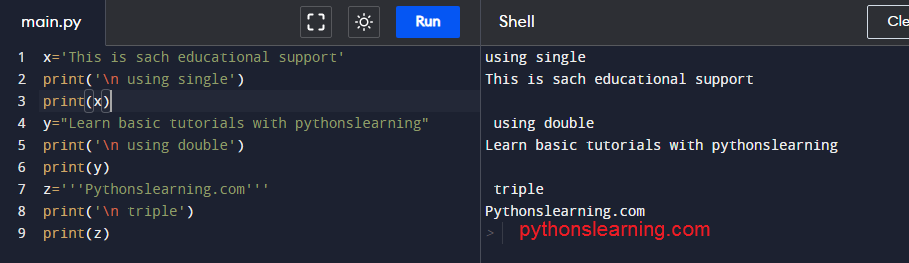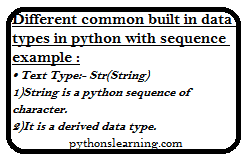string in python

## basic python data types with examples

### Different common built in data types in python with sequence example :Different common built in data types in python with sequence example

• Text Type:- Str(String)
1)String is a python sequence of character.
2)It is a derived data type.

``````x='This is sach educational support'
print('\n using single')
print(x)
y="Learn basic tutorials with pythonslearning"
print('\n using double')
print(y)
z='''Pythonslearning.com'''
print('\n triple')
print(z)``````

Also read : comprehension in python

• Numeric Types:- int, float, complex

Lists in Python can be created by just placing the sequence data inside square brackets.
Unlike Sets, a list doesn’t need a built-in function for the creation of a list.
Note – We know that Sets, the list may contain mutable elements.
1)Int is a integer. It is used to convert a specified value into an integer number.
2)Float is a data type used to return a floating point number from a number or string.
3)Complex is used to convert number or string into complex number.

• Sequence Types:-List,Tuple,Range
1)List is a collection of object. List are ordered in type. It contain arbitrary objects. Elements of a list can accessed by an index. List contain other list as sublists. Lists are in variable in size. Lists are mutable that’s why list can be changed.

``````# Python program to demonstrate
# Creation of List

# Creating a List
List = []
print("Emty list")
print(List)

# Creating a List of numbers
List = [10, 20, 30]
print("\nList of numbers: ")
print(List)

# Creating a List of strings and accessing
# using index
List = ["sach", "Educational", "Support"]
print("\nList Items: ")
print(List)
print(List)

# Creating a Multi-Dimensional List
List = [['Sach', 'Educational'], ['Support']]
print("\nMulti-Dimensional List: ")
print(List)
``````

2)Tuple is an immutable list. Once a tuple is created it cannot be changed. Tuple is analogously to lists. Tuples are faster as compare to list.to protect data from accidental changes use tuples instead of using list. Tuples can be used as keys in dictionaries while list can’t be used in dictionaries.

``````# Creating an empty Tuple
Tuple = ()
print("Initial empty Tuple: ")
print(Tuple)

# Creating a Tuple with string
Tuple1 = ('Sachin', 'Pagar')
print("\nTuple with the use of String: ")
print(Tuple1)

# Creating a Tuple with list
list1 = [1, 2, 4, 5, 6]
print("\nTuple using List: ")
print(tuple(list1))

# Creating a Tuple with the use of built-in function
Tuple1 = tuple('sach')
print("\nTuple with the use of function: ")
print(Tuple1)
``````

3)In Range function elements can be accessed using index values.

Also see : Generator in python
• Mapping Types:-Dict
1)Dict is a Dictionary . It is mutable.keys of dict are arbitrary.
• Set Types:-Set,Frozenset
1)Set is a mutable.add(),remove() like these operations performs on set but it is not possible to do these operations on frozenset. Set do not record element position. Set do not support indexing,slicing, or any other sequence related operations.
2)Frozenset is a immutable
• Boolean Type:-bool
Bool is used to represent the truth value of an expression.
In boolean type there are only two possible values True or False.
• Binary Types:-Bytes,bytearray,memoryview
1)Byte and Byte arrays are used to manipulate binary data in python.These are supported by protocol named memoryview.
2)Memoryview can access the memory of other binary object without copying actual data.

Also see : prime factor in python

Summary :

In this article we saw common built in data types in python with sequence example so about this section you have any query then free to ask me